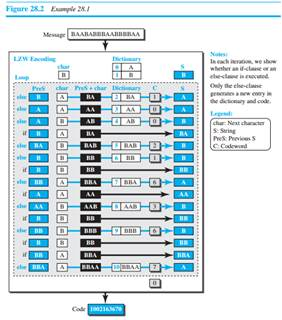Create an Account

Home / Questions / In LZW coding, the message AACCCBCCDDAB is given a Encode the message See Figure 282 b Fin...

In LZW coding, the message AACCCBCCDDAB is given a Encode the message See Figure 282 b Find the compression ratio if we use 8 bits to represent a character and four bits to represent a

In LZW coding, the message “AACCCBCCDDAB” is given.

a. Encode the message. (See Figure 28.2.)

b. Find the compression ratio if we use 8 bits to represent a character and four bits to represent a digit (hexadecimal).Jul 30 2020 View more View LessSubscribe To Get Solution